# Thread: Trigonometric integral

1. ## Trigonometric integral

Can someone help me with this equation thank you. tan^5xsec^4x dx

2.Originally Posted by Link88Can someone help me with this equation thank you. tan^5xsec^4x dx
You need to do three things:

1. Break off a factor of $\displaystyle \sec(x)\tan(x)$
2. Apply the identity $\displaystyle \tan^2(x)=\sec^2(x)-1$, expand and simplify the expressions
3. Then apply the substitution $\displaystyle z=\sec(x)$

You should end up with $\displaystyle \int\left(z^7-2z^5+z^3\right)\,dz$ once you apply the three steps.

3. ##Hello,Originally Posted by Link88Can someone help me with this equation thank you. tan^5xsec^4x dx
$\displaystyle I=\int{tan^5(x)sec^4(x)dx}$
Put tanx = t
so $\displaystyle sec^2(x)=1-t^2$
and $\displaystyle dt=sec^2(x)dx$
hence
$\displaystyle I=\int{t^5(1-t^2)dt}$
$\displaystyle =\int{t^5-t^7 dt}$
you can go ahead4. Don't integrate - balloontegrate!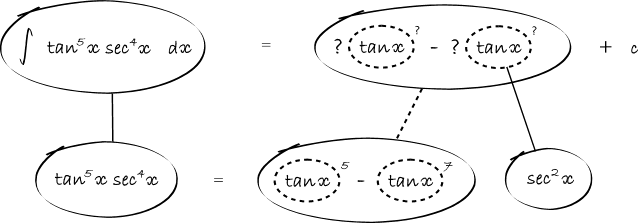(As usual, straight continuous lines differentiate down, integrate up with respect to x. Straight dashed lines with respect to the dashed balloon expression, so that the right hand, triangular network satisfies the chain rule.)

Start by identifying tan x as a possible inner function of the chain rule...Then differentiate for the sake of the chain rule...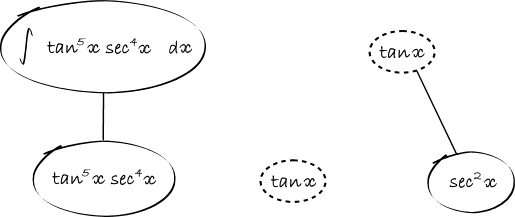Make the lower level of the chain rule shape equal to what you want to integrate...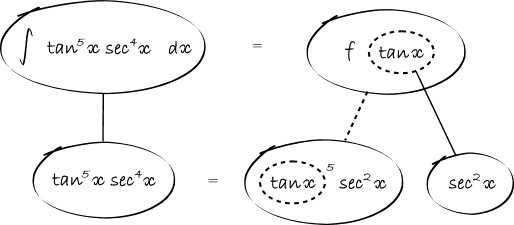Use the trig identity to re-write in terms of the inner function - i.e. so that x appears in the dashed balloon expression if at all, in the big bottom balloon...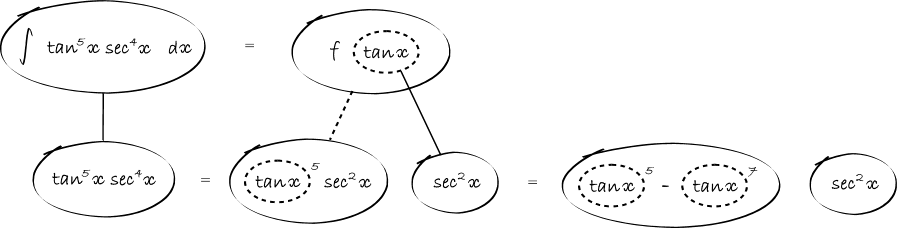Integrate with respect to the dashed balloon, as though it were a variable like x or t ...I.e...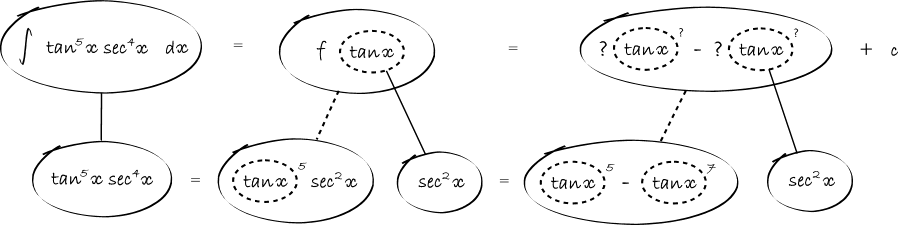Balloon Calculus: worked examples from past papers

#### Search Tags

integral, trigonometric